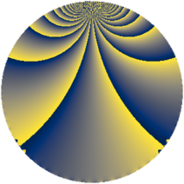# Properties

 Label 429.2.jLevel $429$ Weight $2$ Character orbit 429.j Rep. character $\chi_{429}(122,\cdot)$ Character field $\Q(\zeta_{4})$ Dimension $96$ Newform subspaces $1$ Sturm bound $112$ Trace bound $0$

# Related objects

## Defining parameters

 Level: $$N$$ $$=$$ $$429 = 3 \cdot 11 \cdot 13$$ Weight: $$k$$ $$=$$ $$2$$ Character orbit: $$[\chi]$$ $$=$$ 429.j (of order $$4$$ and degree $$2$$) Character conductor: $$\operatorname{cond}(\chi)$$ $$=$$ $$39$$ Character field: $$\Q(i)$$ Newform subspaces: $$1$$ Sturm bound: $$112$$ Trace bound: $$0$$

## Dimensions

The following table gives the dimensions of various subspaces of $$M_{2}(429, [\chi])$$.

Total New Old
Modular forms 120 96 24
Cusp forms 104 96 8
Eisenstein series 16 0 16

## Trace form

 $$96q + 12q^{6} - 16q^{7} + O(q^{10})$$ $$96q + 12q^{6} - 16q^{7} + 16q^{13} - 16q^{15} - 120q^{16} - 28q^{18} - 24q^{19} + 24q^{24} - 24q^{27} + 56q^{28} + 48q^{31} - 16q^{34} - 16q^{37} + 80q^{40} + 52q^{42} + 4q^{45} - 56q^{46} + 28q^{48} + 4q^{54} + 4q^{57} + 48q^{58} + 4q^{60} - 96q^{61} - 36q^{63} + 20q^{66} - 16q^{67} + 48q^{70} - 16q^{72} - 16q^{73} - 88q^{76} + 80q^{78} + 16q^{79} + 32q^{81} + 52q^{84} - 8q^{85} - 48q^{87} - 16q^{91} - 36q^{93} - 16q^{94} - 108q^{96} - 48q^{97} + O(q^{100})$$

## Decomposition of $$S_{2}^{\mathrm{new}}(429, [\chi])$$ into newform subspaces

Label Dim. $$A$$ Field CM Traces $q$-expansion
$$a_2$$ $$a_3$$ $$a_5$$ $$a_7$$
429.2.j.a $$96$$ $$3.426$$ None $$0$$ $$0$$ $$0$$ $$-16$$

## Decomposition of $$S_{2}^{\mathrm{old}}(429, [\chi])$$ into lower level spaces

$$S_{2}^{\mathrm{old}}(429, [\chi]) \cong$$ $$S_{2}^{\mathrm{new}}(39, [\chi])$$$$^{\oplus 2}$$